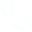# Summary of Common Library Functions in C++ Stl Library

Summary of common library functions in C++ STL library Introduction:

Summary of common library functions in C++ STL library
1. vector , variable-length array, the idea of multiplication
size() returns the number of elements
empty() returns whether it is empty
clear() clear
front()/back() access first element/last element
push_back ()/ pop_back () insert/pop the last element
begin()/end() start element iterator/end element iterator
[]
Supports comparison operations, lexicographically

2. pair< int , int >
first, the first element
second, the second element
Supports comparison operations, with first as the first keyword and second as the second keyword (lexicographical order)

3. string , string
size()/length() returns the length of the string
empty() returns whether it is empty
clear() clear
substr (start index, ( substring length )) return substring
c_str () returns the starting address of the character array where the string is located

4. queue , queue
size() returns the queue length
empty() returns whether it is empty
push() inserts an element to the end of the queue
front() returns the head element of the queue
back() returns the back element
pop() pops the head element of the queue

5. priority_queue, the priority queue, the default is the big root heap
size() returns the queue length
empty() returns whether it is empty
push() inserts an element

top() returns the top element of the heap
pop() pops the top element of the heap
The way to define a small root heap: priority_queue < int , vector < int >, greater< int >> q;

6. stack , stack
size() returns the stack size
empty() returns whether it is empty
push() inserts an element to the top of the stack
top() returns the top element of the stack
pop() pops the top element of the stack

7. deque , double-ended queue
size() returns the queue length
empty() returns whether it is empty
clear() clears the queue
front()/back() returns the head element of the queue
push_back ()/ pop_back () insert/pop the last element
push_front ()/ pop_front () insert/pop the first element
begin()/end() start element iterator/end element iterator
[]

8. set , map , multiset , multimap , based on balanced binary tree (red-black tree), dynamically maintain ordered sequence
size() returns the length
empty() returns whether it is empty
clear() clears the queue
begin()/end() start element iterator/end element iterator
++, -- return predecessor and successor, time complexity O( logn )

set / multiset
insert () inserts a number
find () finds a number
count () returns the number of a certain number
erase ( )
( 1 ) Input is a number x, delete all x O (k + logn )
( 2 ) input an iterator, delete this iterator
lower_bound ( )/ upper_bound ( )
lower_bound (x) returns an iterator of the smallest number greater than or equal to x
upper_bound (x) returns an iterator of the smallest number greater than x
map / multimap
insert () The inserted number is a pair
The input parameter of erase () is a pair or an iterator
find ( )
[] Note that multimap does not support this operation. Time complexity is O ( logn )
lower_bound ( )/ upper_bound ( )

9. unordered_set , unordered_map , unordered_multiset , unordered_multimap , hash table
Similar to the above, the time complexity of adding, deleting, modifying and checking is O ( 1 )
lower_bound ()/ upper_bound () is not supported , iterator's ++, --

10. bitset , pressure bit
bitset <10000> s;
~, &, |, ^
>>, <<
==, !=
[]

count() returns how many 1s there are

any() checks if there is at least one 1
none() to determine whether all 0

set () sets all bits to 1
set (k, v) turns the kth bit into v
reset() turns all bits to 0
flip() is equivalent to ~
flip(k) invert the kth bitContact Us